Next: Schrödinger Wave Equation Up: Quantum Dynamics Previous: Heisenberg Equation of Motion

# Ehrenfest Theorem

We have now introduced all of the basic elements of quantum mechanics. The only thing which is lacking is some rule to determine the form of the quantum mechanical Hamiltonian. For a physical system that possess a classical analogue, we generally assume that the Hamiltonian has the same form as in classical physics (i.e., we replace the classical coordinates and conjugate momenta by the corresponding quantum mechanical operators). This scheme guarantees that quantum mechanics yields the correct classical equations of motion in the classical limit. Whenever an ambiguity arises because of non-commuting observables, this can usually be resolved by requiring the Hamiltonianto be an Hermitian operator. For instance, we would write the quantum mechanical analogue of the classical product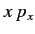, appearing in the Hamiltonian, as the Hermitian product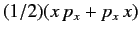. When the system in question has no classical analogue then we are reduced to guessing a form forthat reproduces the observed behavior of the system.

Consider a three-dimensional system characterized by three independent Cartesian position coordinates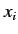(where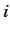runs from 1 to 3), with three corresponding conjugate momenta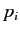. These are represented by three commuting position operators, and three commuting momentum operators, respectively. The commutation relations satisfied by the position and momentum operators are [see Equation (116)]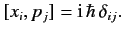(251)

It is helpful to denote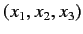as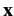and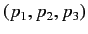as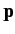. The following useful formulae,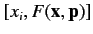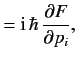(252)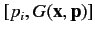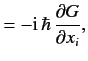(253)

where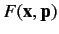and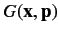are functions that can be expanded as power series, are easily proved using the fundamental commutation relations, (251).

Let us now consider the three-dimensional motion of a free particle of mass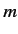in the Heisenberg picture. The Hamiltonian is assumed to have the same form as in classical physics: i.e.,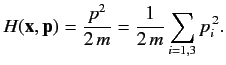(254)

In the following, all dynamical variables are assumed to be Heisenberg dynamical variables, although we will omit the subscriptfor the sake of clarity. The time evolution of the momentum operatorfollows from the Heisenberg equation of motion, (244). We find that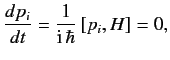(255)

sinceautomatically commutes with any function of the momentum operators. Thus, for a free particle, the momentum operators are constants of the motion, which means that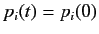at all times(foris 1 to 3). The time evolution of the position operatoris given by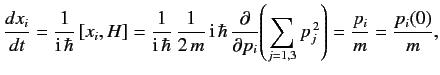(256)

where use has been made of Equation (252). It follows that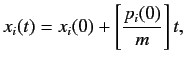(257)

which is analogous to the equation of motion of a classical free particle. Note that even though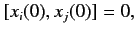(258)

where the position operators are evaluated at equal times, thedo not commute when evaluated at different times. For instance,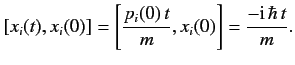(259)

Combining the above commutation relation with the uncertainty relation (83) yields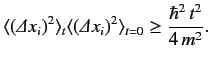(260)

This result implies that even if a particle is well-localized at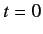, its position becomes progressively more uncertain with time. This conclusion can also be obtained by studying the propagation of wavepackets in wave mechanics.

Let us now add a potential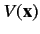to our free particle Hamiltonian: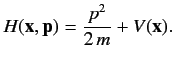(261)

Here,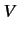is some (real) function of theoperators. The Heisenberg equation of motion gives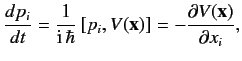(262)

where use has been made of Equation (253). On the other hand, the result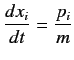(263)

still holds, because theall commute with the new term,, in the Hamiltonian. We can use the Heisenberg equation of motion a second time to deduce that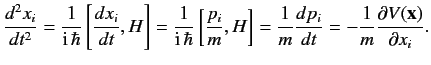(264)

In vectorial form, this equation becomes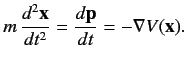(265)

This is the quantum mechanical equivalent of Newton's second law of motion. Taking the expectation values of both sides with respect to a Heisenberg state ket that does not evolve in time, we obtain the so-called Ehrenfest theorem: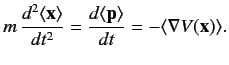(266)

When written in terms of expectation values, this result is independent of whether we are using the Heisenberg or Schrödinger picture. By contrast, the operator equation (265) only holds ifandare understood to be Heisenberg dynamical variables. Note that Equation (266) has no dependence on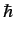. In fact, it guarantees to us that the centre of a wavepacket always moves like a classical particle.Next: Schrödinger Wave Equation Up: Quantum Dynamics Previous: Heisenberg Equation of Motion
Richard Fitzpatrick 2013-04-08